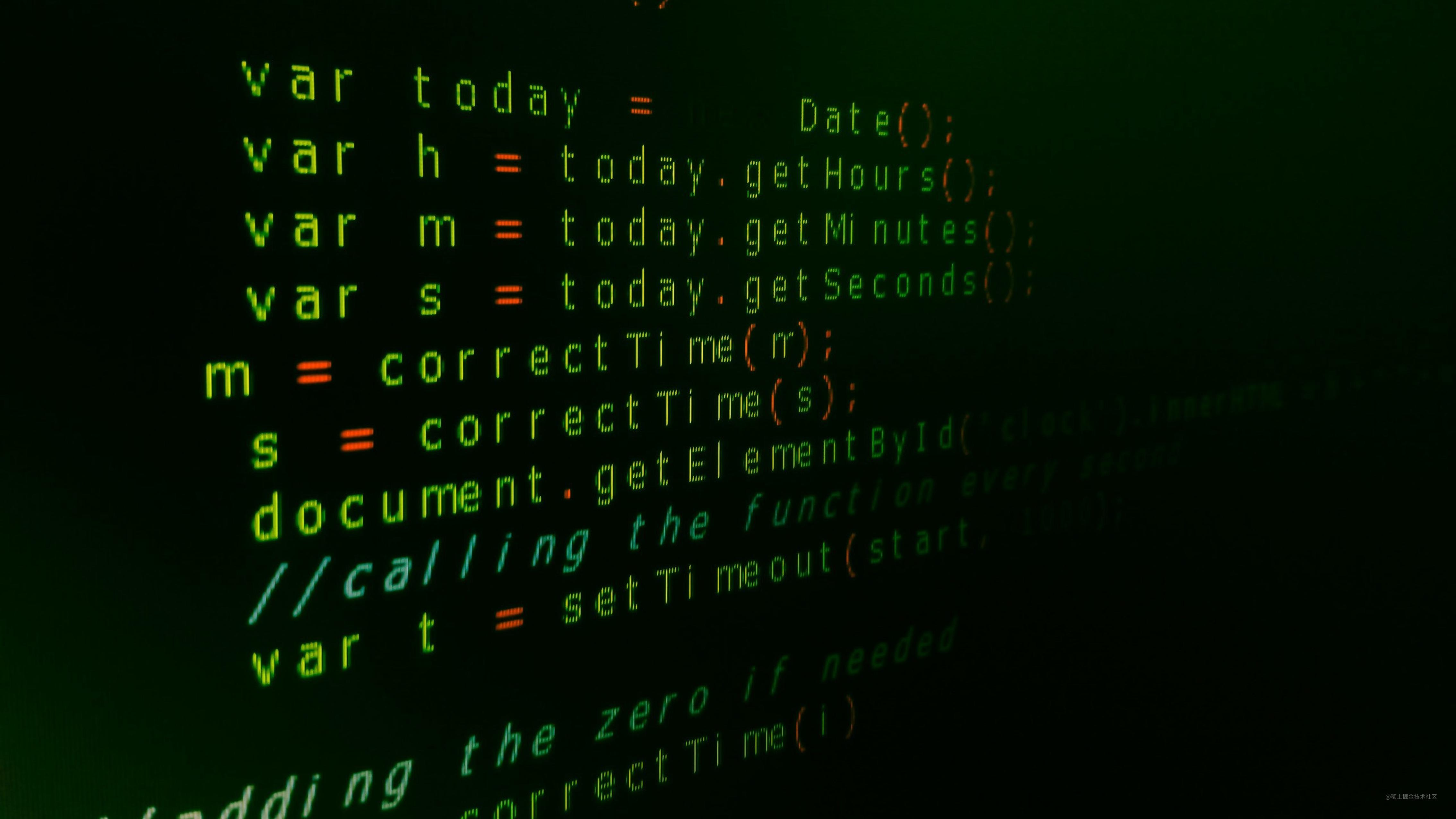# 什么是纯函数，JavaScript函数式编程的基础• 它应始终返回相同的值。不管调用该函数多少次，无论今天、明天还是将来某个时候调用它。
• 自包含（不使用全局变量）。
• 它不应修改程序的状态或引起副作用（修改全局变量）。

## 第一个条件：应始终返回相同的值

``````Math.cos(0) // 1

``````const numberOfApples = 5;
const applesBought = 5;
const add = (num1, num2) => num1 + num2;
const totalApples = add(numberOfApples, applesBought) // 10
const totalApples = add(numberOfApples, applesBought) // 10
// ... one month later
const totalApples = add(numberOfApples, applesBought) //10

``````Math.random() // 返回一个随机数
Math.random() // 返回一个不同的随机数
// 一个月后
Math.random() // 返回一个不同的随机数

## 第二标准：自包含

``````const numberOfApples = 5
const applesBought = 5
const addApplesToTotal = (num1) => numberOfApples + num1;
const totalApples = addApplesToTotal(applesBought); // 10

## 第三个条件：它不应修改程序的状态或引起副作用

``````let totalApples = 5
const applesBought = 5
const addApplesToTotal = () => {
totalApples += applesBought
};

• 它正在访问外部作用域，因此它不是自包含的。
• 它正在引起副作用，因为它改变了 `totalApples` 的值。

• 纯函数不那么复杂
• 更容易调试 🐛
• 易于组合
• 易于并行化